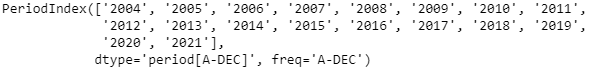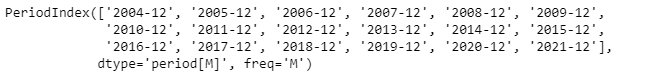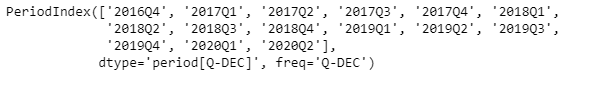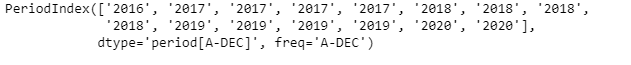# Python | Pandas PeriodIndex.asfreq

Python is a great language for doing data analysis, primarily because of the fantastic ecosystem of data-centric python packages. Pandas is one of those packages and makes importing and analyzing data much easier.

Pandas` PeriodIndex.asfreq()` function convert each element of the given PeriodIndex object to the specified frequency.

Syntax : PeriodIndex.asfreq(freq=None, how=’E’)

Parameters :
freq : a frequency
how : ‘E’, ‘END’, or ‘FINISH’ for end, ‘S’, ‘START’, or ‘BEGIN’ for start.

Return : PeriodIndex with the new frequency

Example #1: Use `PeriodIndex.asfreq()` function to convert the frequency of the given PeriodIndex object to the specified time series frequency.

 `# importing pandas as pd ` `import` `pandas as pd ` ` `  `# Create the PeriodIndex object ` `pidx ``=` `pd.PeriodIndex(start ``=``'2004-11-11 02:45:21 '``,  ` `                ``end ``=``'2021-5-21 8:45:29'``, freq ``=``'Y'``) ` ` `  `# Print the PeriodIndex object ` `print``(pidx) `

Output :Now we will use the `PeriodIndex.asfreq()` function to convert the time series frequency of the given PeriodIndex object to the specified frequency.

 `# convert the frequency ` `# 'M' stands for monthly frequency ` `pidx.asfreq(``'M'``) `

Output :As we can see in the output, the `PeriodIndex.asfreq()` function has converted the frequency of the given PeriodIndex object to the specified frequency.

Example #2: Use `PeriodIndex.asfreq()` function to convert the frequency of the given PeriodIndex object to the specified time series frequency.

 `# importing pandas as pd ` `import` `pandas as pd ` ` `  `# Create the PeriodIndex object ` `pidx ``=` `pd.PeriodIndex(start ``=``'2016-10-12 11:12:02'``,  ` `             ``end ``=``'2020-04-12 11:32:12'``, freq ``=``'Q'``) ` ` `  `# Print the PeriodIndex object ` `print``(pidx) `

Output :Now we will use the `PeriodIndex.asfreq()` function to convert the time series frequency of the given PeriodIndex object to the specified frequency.

 `# convert the frequency ` `# 'Y' stands for yearly frequency ` `pidx.asfreq(``'Y'``) `

Output :As we can see in the output, the `PeriodIndex.asfreq()` function has converted the frequency of the given PeriodIndex object to the specified frequency.

My Personal Notes arrow_drop_upCheck out this Author's contributed articles.

If you like GeeksforGeeks and would like to contribute, you can also write an article using contribute.geeksforgeeks.org or mail your article to contribute@geeksforgeeks.org. See your article appearing on the GeeksforGeeks main page and help other Geeks.

Please Improve this article if you find anything incorrect by clicking on the "Improve Article" button below.

Article Tags :

Be the First to upvote.

Please write to us at contribute@geeksforgeeks.org to report any issue with the above content.